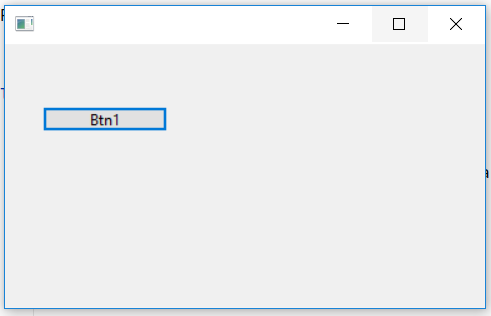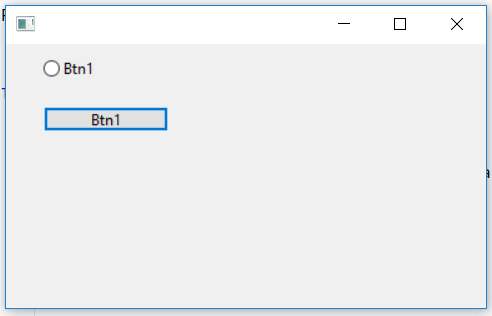Related Articles

# wxPython – Show hidden Radio Button

• Last Updated : 26 Jun, 2020

In this article we will learn how can we show a hidden radio button. In order to unhide/show a radio button we can use Show() function. Show() function can be used to do both show as well as hide the radio button. Show() function takes show boolean parameter which, If True displays the window. Otherwise, hides it.

Parameters:

ParameterInput TypeDescription
showboolIf True displays the window. Otherwise, hides it.

Return Type: bool

Returns: True if the window has been shown or hidden or False if nothing was done because it already was in the requested state.

Code Example:

 `import` `wx`` ` `APP_EXIT ``=` `1`` ` ` ` `class` `Example(wx.Frame):`` ` `    ``def` `__init__(``self``, ``*``args, ``*``*``kwargs):``        ``super``(Example, ``self``).__init__(``*``args, ``*``*``kwargs)`` ` `        ``self``.InitUI()``    ``def` `InitUI(``self``):``        ``self``.pnl ``=` `wx.Panel(``self``)``         ` `        ``# create radio button at position (30, 10)``        ``self``.rb1 ``=` `wx.RadioButton(``self``.pnl, label ``=``'Btn1'``, pos ``=``(``30``, ``10``), size ``=``(``100``, ``20``))``         ` `        ``# create button``        ``self``.btn ``=` `wx.Button(``self``.pnl, label ``=``'Btn1'``, ``                           ``pos ``=``(``30``, ``50``), size ``=``(``100``, ``20``))`` ` `        ``# hide radio button``        ``self``.rb1.Hide()`` ` `        ``# bind event function``        ``self``.btn.Bind(wx.EVT_BUTTON, ``self``.Onclick)``     ` `    ``def` `Onclick(``self``, e):``            ``# show hidden radio button``            ``self``.rb1.Show()`` ` `def` `main():``    ``app ``=` `wx.App()``    ``ex ``=` `Example(``None``)``    ``ex.Show()``    ``app.MainLoop()`` ` ` ` `if` `__name__ ``=``=` `'__main__'``:``    ``main()`

Output Window:before clickin buttonafter clicking button

Attention geek! Strengthen your foundations with the Python Programming Foundation Course and learn the basics.

To begin with, your interview preparations Enhance your Data Structures concepts with the Python DS Course. And to begin with your Machine Learning Journey, join the Machine Learning – Basic Level Course

My Personal Notes arrow_drop_up